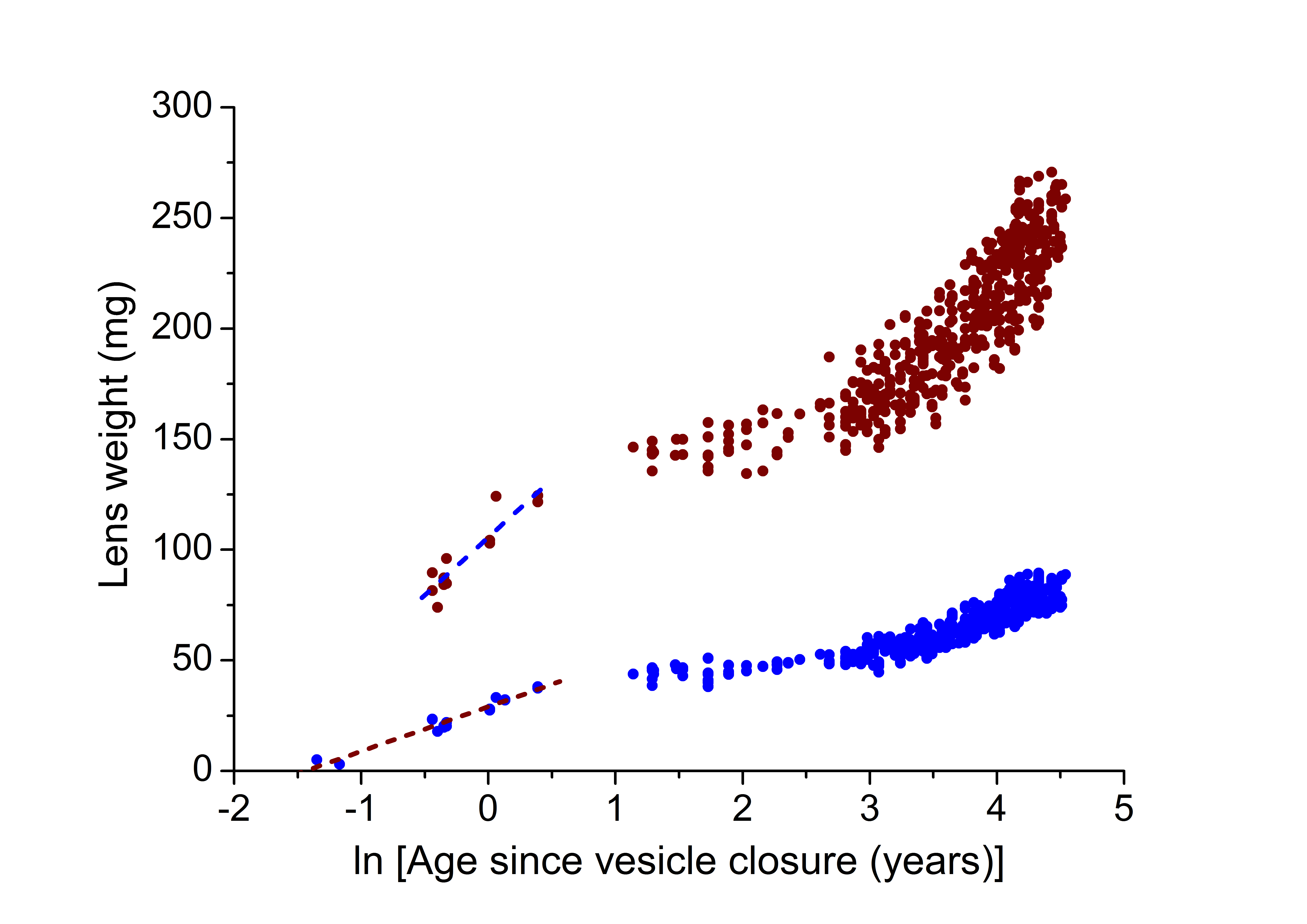Figure 4 of Mohamed, Mol Vis 2018; 24:867-874.

Figure 4. Semilogarithmic analyses of lens Wet (brown symbols) and Dry (blue symbols) weights as a function of age. Regression analysis of all less than 2 year data yielded straight lines with wet weight = 105.65 (±2.32) + 52.36 (±7.0) × ln [Av] (R2=0.85; p<0.0001; n=12) and dry weight = 29.02 (±0.65) + 20.16 (±1.67) × ln [Av] (R2=0.95; p<0.0001; n=16).